# How to Count Cells Greater Than a Specific Value in Excel

This post will guide you how to count the number of cells greater than a particular numeric value in a given range cells using a formula in Excel 2013/2016. How do I count cells that are greater than a specific value through VBA Macro in Excel.

## Count Cells Greater Than a Specific Value

Assuming that you want to count cells that have values greater than a particular number (70).In this case, you can use the COUNTIF function to count the number of cells in range B2:B6 that have a value greater than the value (70) or in cell D1. Using the following formula:

=COUNTIF(B2:B6,”>=”&D1)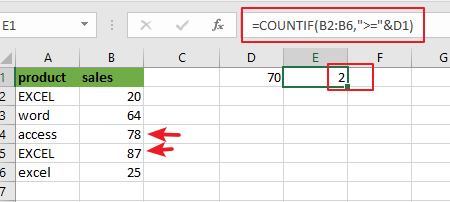Or you can also use the COUNTIF function to count the number of cells that contain a value of greater than a specific value (70), which is directly entered into the formula, type:

=COUNTIF(B2:B6,”>70″)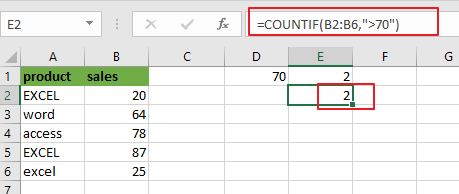Note: for the above formula, B2:B6 is a range of cells that you want to use, and “`>70`” is the criteria above which you want to count. You also need to know that the COUNTIF function is not case-sensitive.

Now Let’s see the syntax of the COUNTIF Function:

The COUNTIF function is a built-in function. It can be used to do several calculations to find out exactly how many cells in a given range that have a value of greater than or less than or equal to the value that you have specified.

=COUNTIF (Range, Criteria)

This function has two arguments, range and criteria, which are required to get the exact result.

• Range: when one or more than one cell is taken into account for counting operation, then this is regarded as a range.
• Criteria: it is a specific condition that you want to specify to let the function know which cells you want to take into account for counting. It could be any text string, number, cell reference or an array list.

Note: you need to know that the COUNTIF function is not case sensitive. And if you want to count the number of cells in a range with case-sensitive, you need to use EXACT function.

## Count Cells Greater Than a Specific Value Through VBA

To count the number of cells in the range that contain numeric values greater than a specific value, and you can use the Excel VBA Macro to achieve the same result. Just do the following steps:

Step1# open your excel workbook and then click on “Visual Basic” command under DEVELOPER Tab, or just press “ALT+F11” shortcut.Step2#  then the “Visual Basic Editor” window will appear.

Step3# click “Insert” ->”Module” to create a new module.Step4# paste the below VBA code into the code window. Then clicking “Save” button.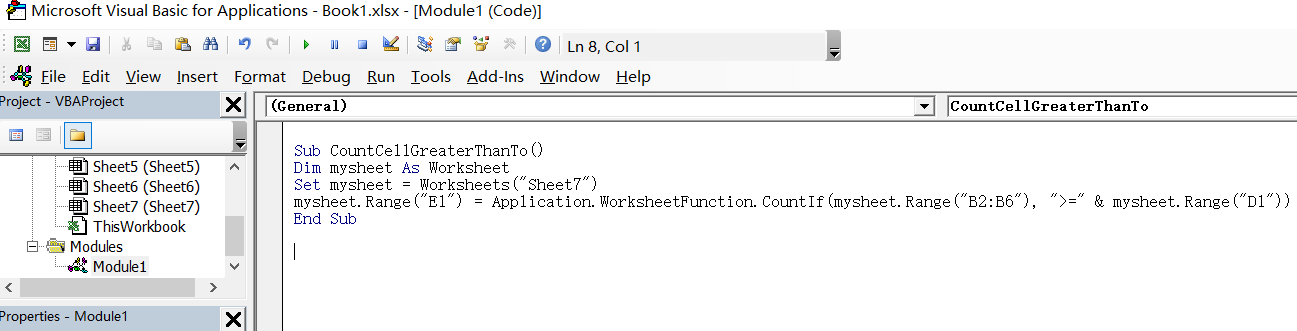```Sub CountCellGreaterThanTo()

Dim mysheet As Worksheet

Set mysheet = Worksheets("Sheet7")

mysheet.Range("E1") = Application.WorksheetFunction.CountIf(mysheet.Range("B2:B6"), ">=" & mysheet.Range("D1"))

End Sub```

Step5: back to the current worksheet, click on `Macros` button under `Code` group. then click `Run` button.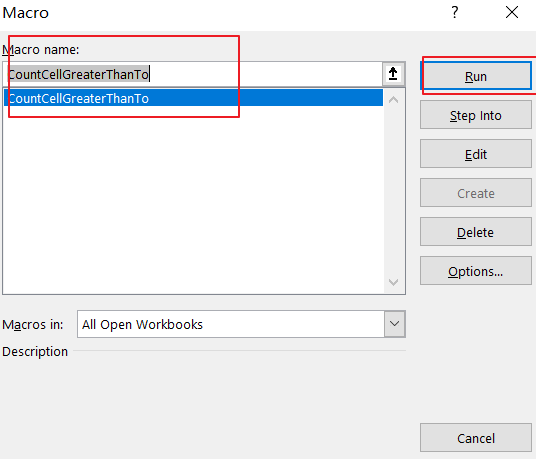Step6: let’s see the result: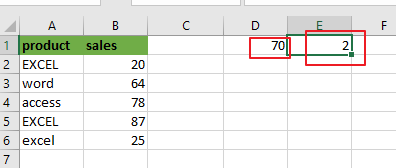Note:

Cell` E1` is the output range, you need to change the cell reference E1 as you need in the VBA code.

`Sheet7` is the worksheet name, and you need to change the name of “mysheet” object variable as you need.

`B2:B6` is the range from which you want to count cells that are greater than a particular cell value in cell D1.

`D1` is a specific value that you want to count if a cell’s value is greater than to the value in Cell D1.

If you want to count the cells that are greater than a specific value with the value entered directly into the VBA code, you can use the following VBA code:

```Sub CountCellGreaterThanTo()

Dim mysheet As Worksheet

Set mysheet = Worksheets("Sheet7")

mysheet.Range("E1") = Application.WorksheetFunction.CountIf(mysheet.Range("B2:B6"), ">=70")

End Sub```

### Related Functions

• Excel COUNTIF function
The Excel COUNTIF function will count the number of cells in a range that meet a given criteria. This function can be used to count the different kinds of cells with number, date, text values, blank, non-blanks, or containing specific characters, etc.= COUNTIF (range, criteria)…
• Excel EXACT function
The Excel EXACT function compares if two text strings are the same and returns TRUE if they are the same, Or, it will return FALSE. The syntax of the EXACT function is as below:= EXACT (text1,text2)…